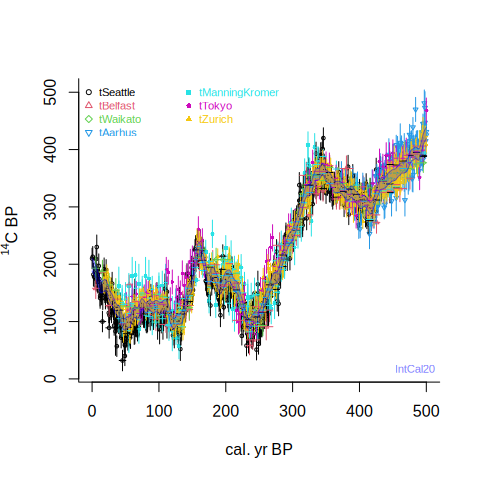# 2. plots

We can also plot a calibration curve:

draw.ccurve()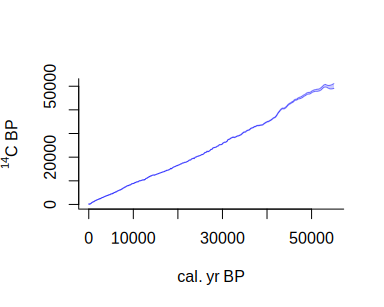or zoom in to between AD 1600 and 2000 (using the BCAD scale):

draw.ccurve(1600, 1950, BCAD=TRUE)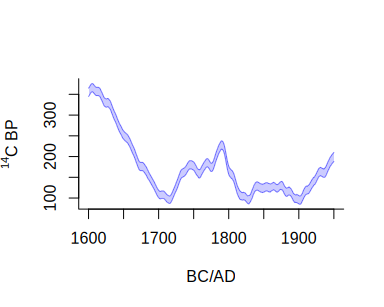Interesting things happened after 1950, as can be seen by adding a postbomb curve:

draw.ccurve(1600, 2020, BCAD=TRUE, cc2='nh1')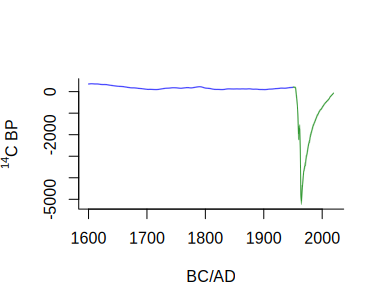The postbomb curve dwarves the IntCal20 curve, so we could also plot both on separate vertical axes:

draw.ccurve(1600, 2020, BCAD=TRUE, cc2='nh1', add.yaxis=TRUE)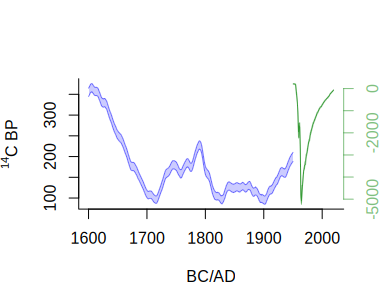We can also visualise the data underlying parts of the IntCal calibration curve, for example from 500 to 0 cal BP:

intcal.data(0, 500)